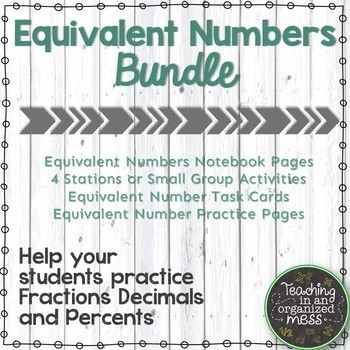# Converting Fractions, Decimals, and Percents -- Equivalent Number BUNDLESubject
Resource Type
Product Rating
4.0
2 Ratings
File Type

Compressed Zip File

Be sure that you have an application to open this file type before downloading and/or purchasing.

19 MB|50+
Share
4 Products in this Bundle
4 products
1. 20 task cards to help students with equivalent forms of fraction decimals and percents. The are various tasks where students will find equivalent forms of fractions, decimals, and percents in real-world situations and models. These task cards will help students with TEKS 6.4E, 6.4F, 6.4G, and 6.5
2. These activities help students understand equivalent fraction decimals and percents and practice converting between the forms of equivalent numbers. There are 4 different activities that can be used for small group for teaching or interventions. The activities can also be used for students in statio
3. Introduce students to percents as they relate to fractions and decimals. The notebook pages use models to help students connect equivalent numbers. This resources also provides students with the steps to convert between fractions, decimals, and percents. There is lot of practice included that can
4. Students will practice converting between fractions, decimals, and percents in a fun, engaging way--Escape Room! There are 6 different challenges for students to practice finding equivalent forms of fractions, decimals, and percents and using models. This is a great way to practice and assess at the
Bundle Description
Save over 20% by purchasing this BUNDLE of equivalent number resources. It focuses on converting between fractions, decimals, and percents. It comes with interactive notebook pages, practice pages, exit tickets, stations, and task cards. The Fraction, Decimal, Percent Escape Room was just added!

Total Pages
50+